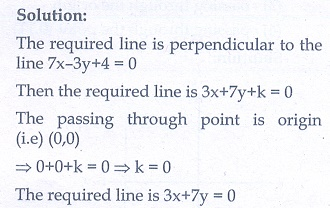# Chapter 5: Coordinate Geometry - Online Test

Q1. The area of triangle formed by the points (−5,0) , (0,−5) and (5,0) is
Explaination / Solution: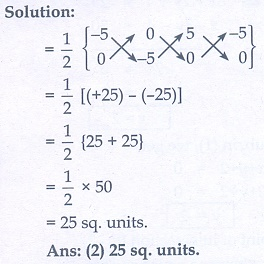Q2. A man walks near a wall, such that the distance between him and the wall is 10 units. Consider the wall to be the Y axis. The path travelled by the man is
Explaination / Solution:Q3. The straight line given by the equation x = 11 is
Explaination / Solution: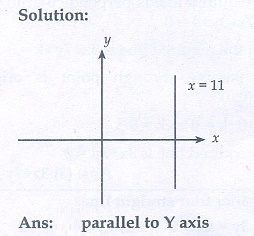Q4. If (5,7), (3,p) and (6,6) are collinear, then the value of p is
Explaination / Solution: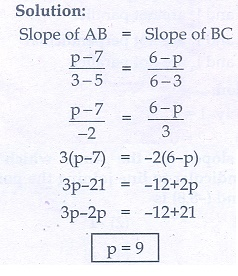Q5. The point of intersection of 3xy = 4 and x + y = 8 is
Explaination / Solution: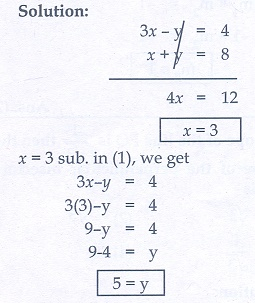Q6. The slope of the line joining (12, 3) , (4,a) is 1/8. The value of ‘a’ is
Explaination / Solution:Q7. The slope of the line which is perpendicular to a line joining the points (0,0) and (–8,8) is
Explaination / Solution:Q8. If slope of the line PQ is 1/√3 then slope of the perpendicular bisector of PQ is
Explaination / Solution:Q9. If A is a point on the Y axis whose ordinate is 8 and B is a point on the X axis whose abscissae is 5 then the equation of the line AB is
Explaination / Solution:Q10. The equation of a line passing through the origin and perpendicular to the line 7x − 3y + 4 = 0 is
Explaination / Solution: